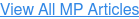## Search Mechanical Products Website# Mechanical Products Blog

Whenever a piece of electrical equipment is first energized, transients will occur to some degree in the device electrical, thermal and perhaps, mechanical characteristics.  Although overcurrent transients are present in nearly all types of electrical apparatus, major overcurrent transients typically occur in transformers and electric motors.  We shall examine each of these two start-up transient overcurrent situations in some detail.

Transformers are magnetically coupled windings which transform the voltage levels of the windings approximately by the ratio of the number of turns within the windings.  The coupling medium or magnetic core in a power transformer is ferromagnetic steel, which can support much larger levels of induction (magnetic flux density) that can be obtained in non-magnetic materials, such as air.  It is this core material which is responsible for the inrush phenomenon in transformers.

An idealizing two-winding transformer construction and its electrical equivalent circuit are shown in Figure 2.17.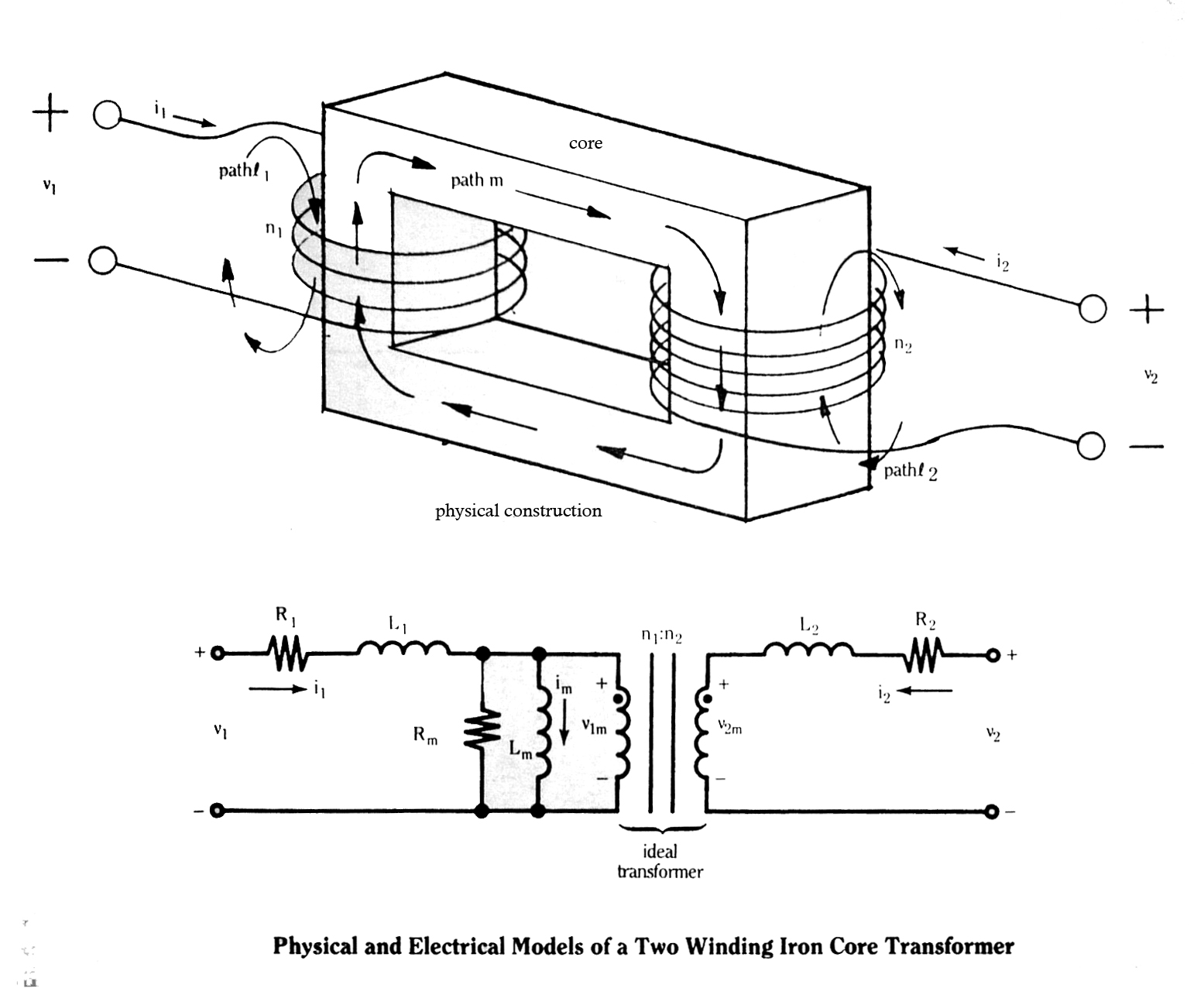The resistance elements, R1 and R2, represent the ohmic resistances of the two windings, and the winding inductance elements, L1 and L2, represent the inductances of the leakage flux paths, 1 and 2, respectively, which are mostly in air.  Fluxes in these two paths, φ₰1 and φ₰2, are termed leakage fluxes, since they do not mutually couple the two windings.  The mutual or coupling flux path, m, which is almost entirely through the core medium, passes the mutual or magnetizing flux, φm.  The magnetization curve for this mutual path is shown in figure 2.18.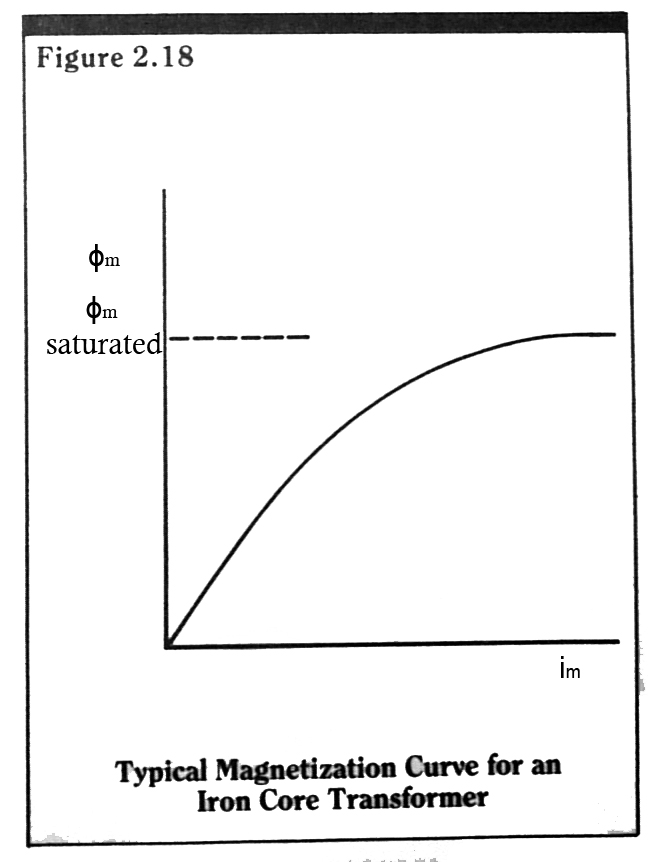The mutual flux, φm, is proportional to a magnetizing current, im, up to a saturation limit, φm sat.  In terms of a flux density value, this limiting value of magnetic induction within the core is approximately 2.1 Teslas for transformer steel.

The nonlinear inductance term, Lm, represents the magnetizing characteristics, of the mutual path, m, and the two coupled windings. The equivalent magnetizing loss resistor, Rm, accounts for the energy that is lost in magnetizing the core material due to hysteresis and eddy current effects.

The remaining component in the equivalent circuit of Figure 2.17 is an ideal transformer, which transforms the internal terminal voltages, vm1 and vm2, exactly by the turns ratio, n1/n2.

Consider now the simple case of energizing the transformer primary (side 1 in Figure 2.17) with a sinusoidal voltage.  Assume, again for simplicity, that the transformer secondary is unloaded, that we can neglect the core loss due to Rm, and that there is no residual flux within the core medium from previous operations.  The equivalent circuit for this situation is shown in Figure 2.19.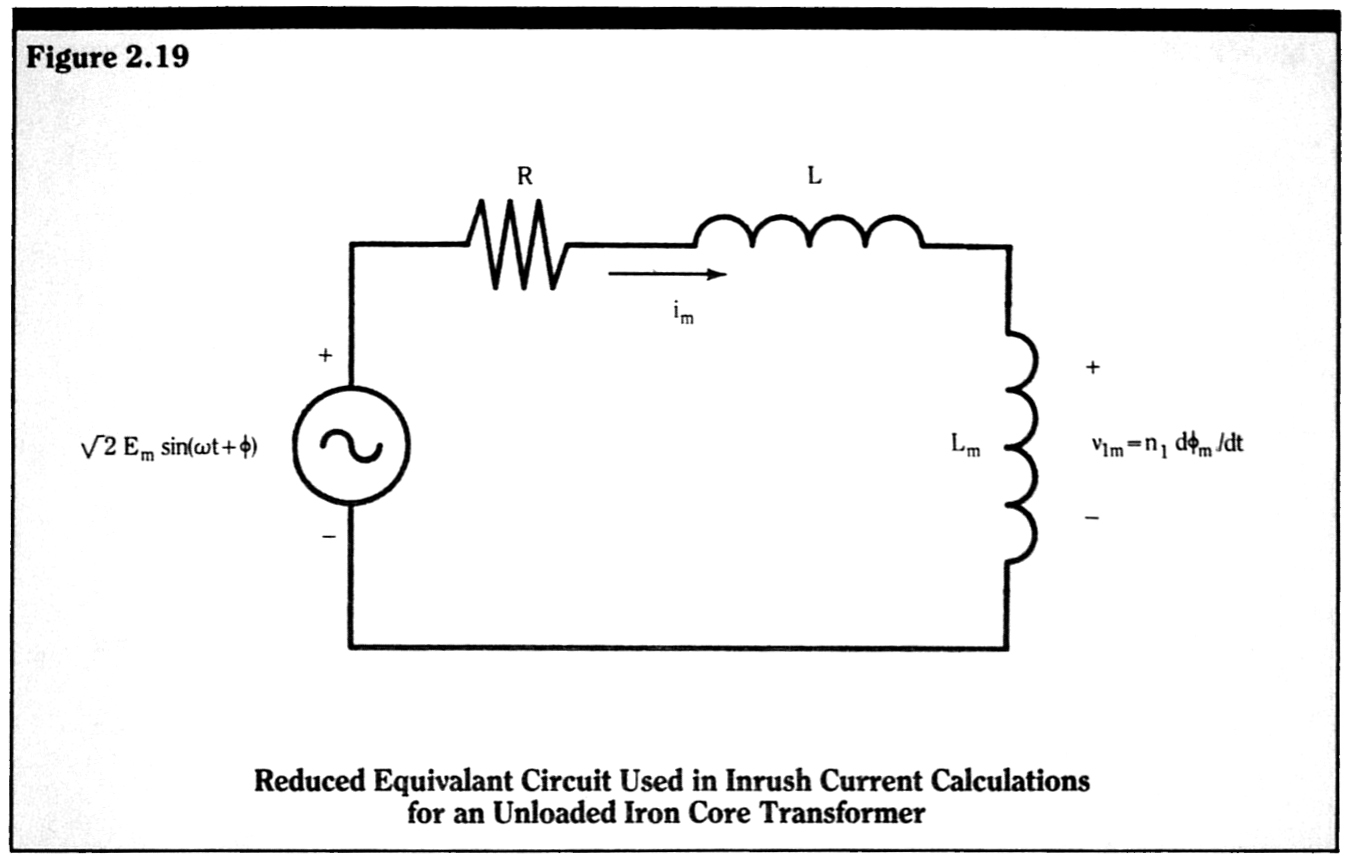The series terms, R and L, account for both the primary winding resistance and the leakage inductance, and any source side line resistance and inductance.  The source voltage phase angle, φ, is again the switching angle at t=0.  In general, the magnetizing inductance Lm, is much greater than the leakage-line inductance, L.  This inductance is so large that it will drop almost the entire value of the source voltage, Es.  For t 0, we thus have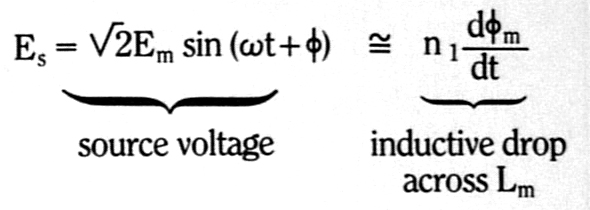Or, upon integrating,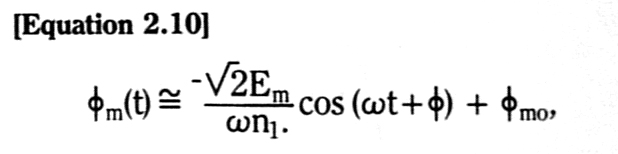where φmo is a constant DC flux.  Since we have assumed that there is no residual flux (that is, φm (0) =0), we must have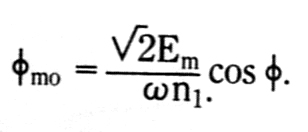For a complete solution, we then have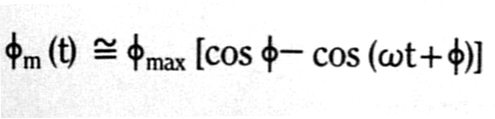where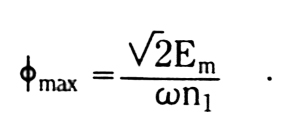As a function of switching angle φ, the worst case is clearly that of φ=0o or 180o, that is, switching at zero crossing of the excitation voltage.  For the case of φ = 0o, we have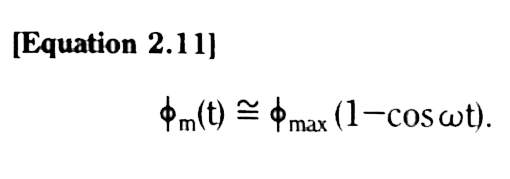In actuality, the DC flux term, φmo (the constant term in Equations 2.10 and 2.11) would decay exponentially with a time constant, (<Lm> + L) /R, where <Lm> is an average, effective value for the nonlinear, time-varying magnetizing inductance, Lm.  Note that, since Lm is large, this time constant for a low loss circuit could be quite long, of the order of tenth of seconds.

A transient magnetizing flux, φm (t), of the form of Equation 2.11, would induce a very large value of inrush current, with peaks of up to ten to twenty, or even more, times the full load steady-state current peaks.  This is due to the fact that the peaks of the transient magnetizing flux variation are approximately twice the steady-state flux peaks.  Since transformers are designed so that steady-state magnetizing flux peaks are approximately at the knee of the magnetization curve (see Figure 2.18), these double value peak fluxes are usually well into the saturation region of the core material magnetizing characteristic.  The saturation effect is shown graphically in Figure 2.20 for the flux variation of Equation 2.11.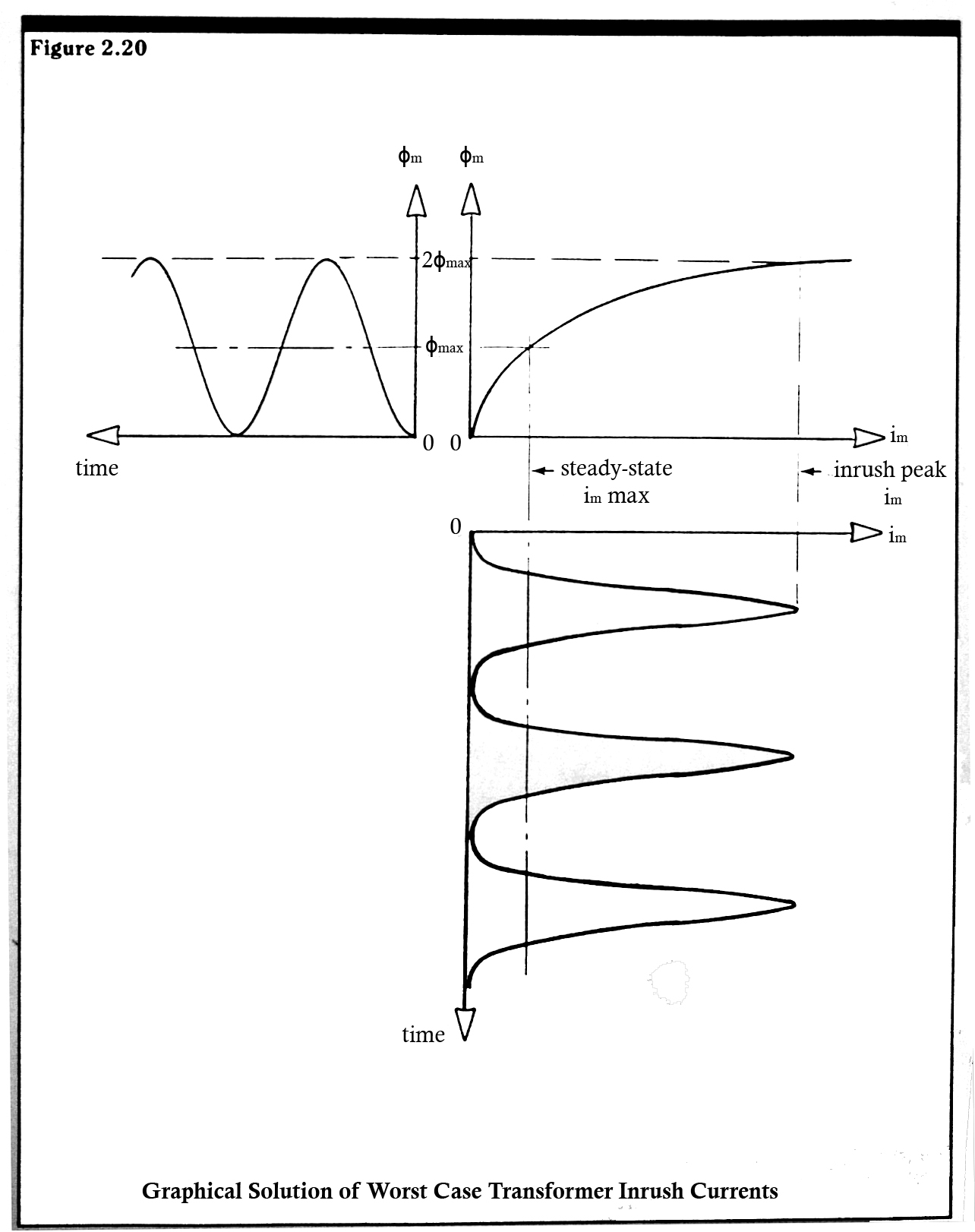Clearly the required magnetizing current, when the magnetizing flux is close to its saturation value, can be many times the rated current of the transformer.

An actual, measured set of inrush waveforms for a small, single phase, 550VA power supply transformer with an open secondary winding is shown in Figure 2.21a.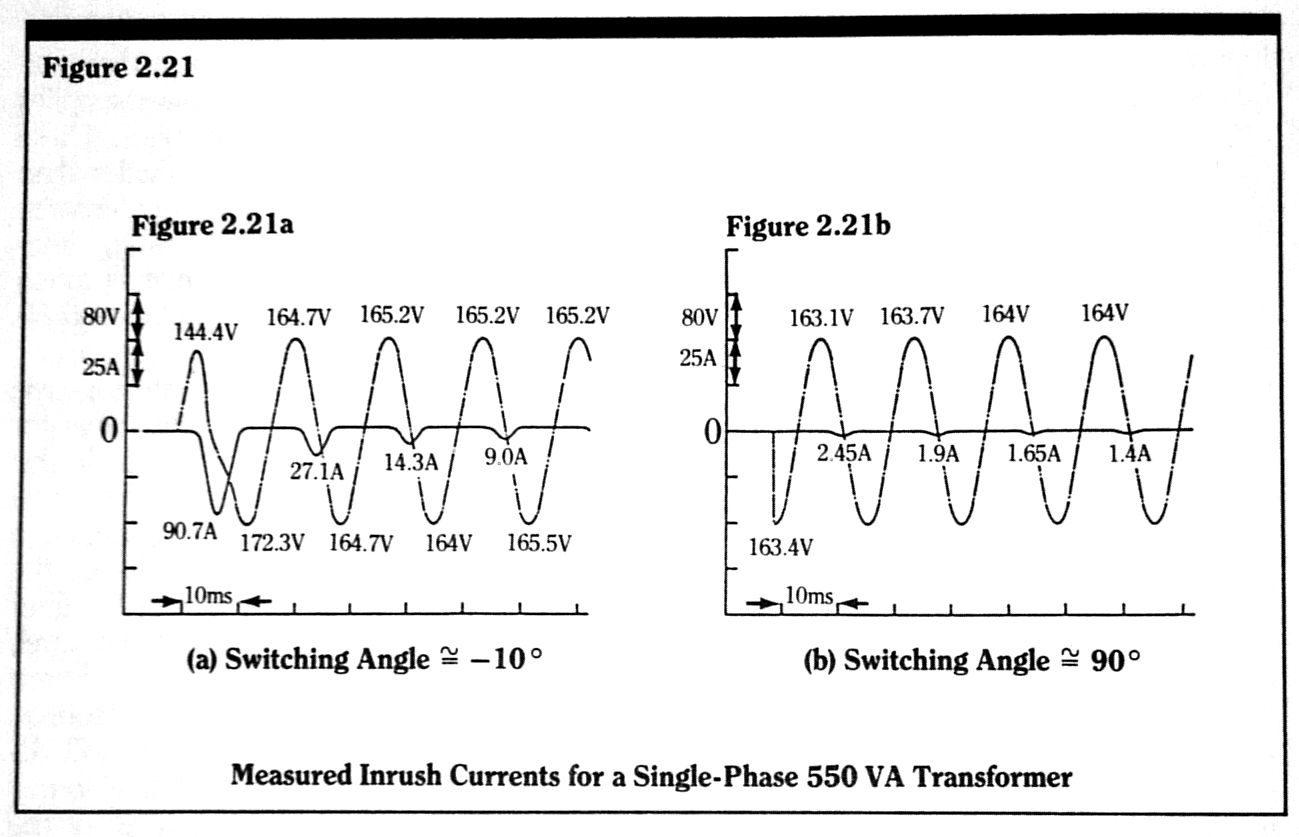As can be seen from the voltage waveform, the switching angle for this case is approximately -10o.  The rated 120V primary side currents for this transformer is 5 amps RMS.  Note that the first peak of the inrush current is approximately 90 amps, 18 times the rated RMS value.  Also, note that the current peaks have not fallen to their steady-state value, approximately 1.5 amps, even after four electrical cycles from the initial switch closing.  Figure 2.21b shows the results for the same transformer, but with a switching angle of approximately 90o.  Note the almost complete absence of inrush current.  Similar inrush phenomena occur in three-phase transformer banks.

Electric Motors – All types of DC and AC electric motors, for a fixed source voltage, draw larger values of starting current than full load running current.  This is because, at start-up, there is no induced winding back emf or speed voltage to oppose the flow of winding current.  At a condition of zero speed, there is only the winding impedance to limit the flow of startup or locked rotor current.  If the motor should stall at a locked rotor condition, the resultant level of steady-state locked rotor current is generally of sufficient magnitude to cause permanent damage to the motor windings.  If the motor can accelerate to its design operating speed however, the thermal mass of the machine can easily handle the excess heat generated by the overcurrent during a run-up period of reasonable extent.

Locked motor currents are specified by the manufacturer.  Taken from the data of a single manufacturer, the range of locked rotor to full load current ratios for single-phase and three-phase 60 Hz motors, given in Figures 2.25 and 2.26, is typical for all such machines, industry wide.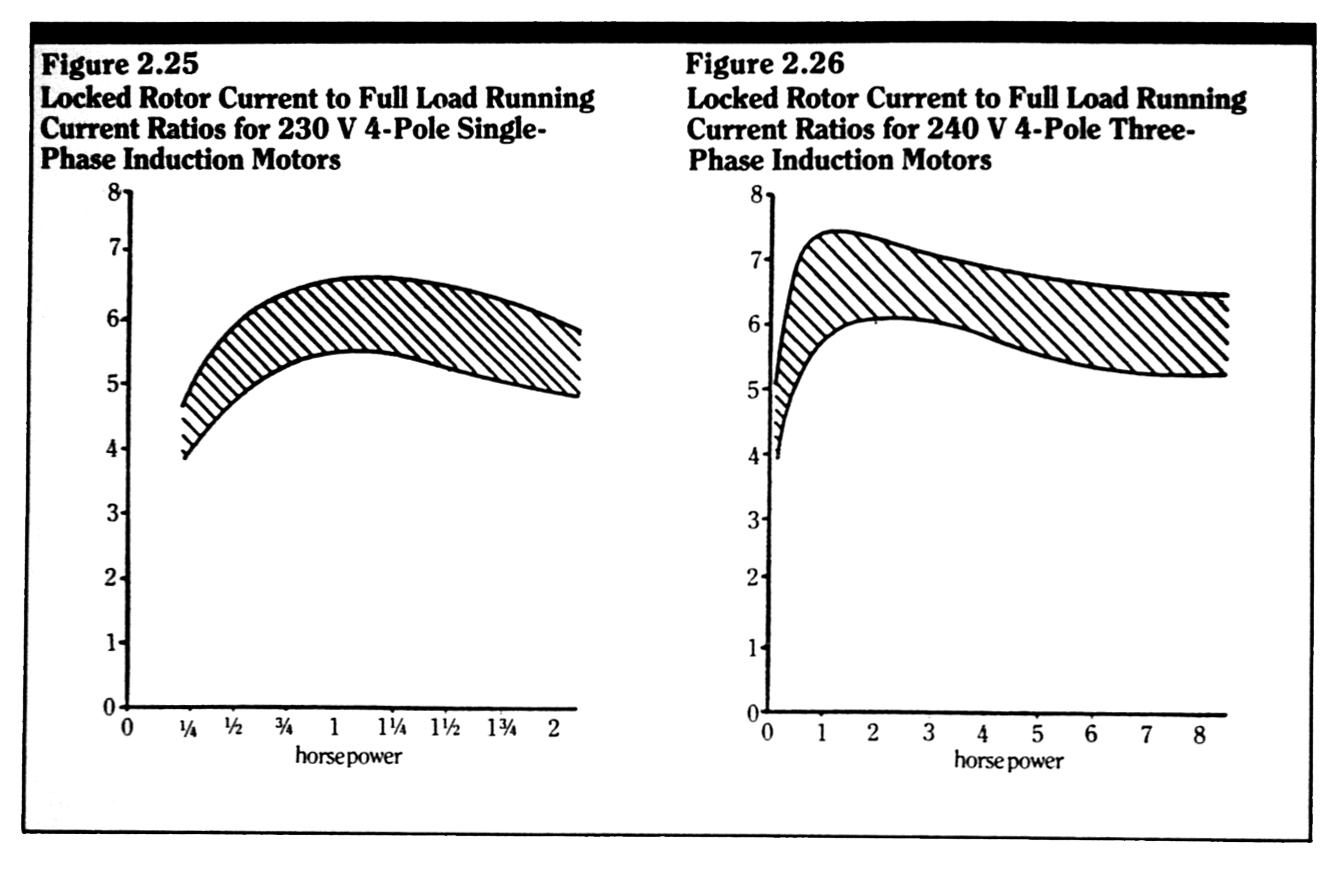For machines of approximately one horsepower or greater, the winding impedances of DC motors are generally so low that auxiliary means, such as the insertion of temporary series resistances, must be used to limit the starting currents to manageable values.  Similar starting methods can be used for AC machines as well.  The generic term, soft-start, is used to describe any auxiliary means to limit the inrush current of a motor.  The term also applies to auxiliary means for limiting start-up currents for other types of electrical apparatus.

The duration of start-up current for motors depends on the type and size of mechanical load that the motor is driving.  The run-up period of an unloaded single-phase motor (less than one horsepower) is less than one second.  But a fully loaded machine, such as a furnace blower, can take several seconds to come up to rated speed.  For a particular application, a safe approximation is to assume that the locked rotor current flows for 80% of the run-up period, and tails off linearly to the running current during the remaining 20%.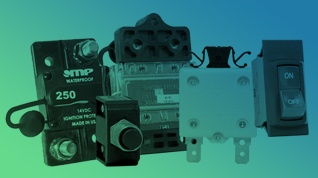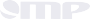#### Product Catalog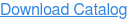# Mechanical ProductsResources

Download the Circuit Breaker Selection Guide.  Covering ratings of 0.5 – 300 amps, the selection guide is a summary overview of the Series of circuit breakers made by MP.  For further details, the individual Series Data Sheets may be downloaded.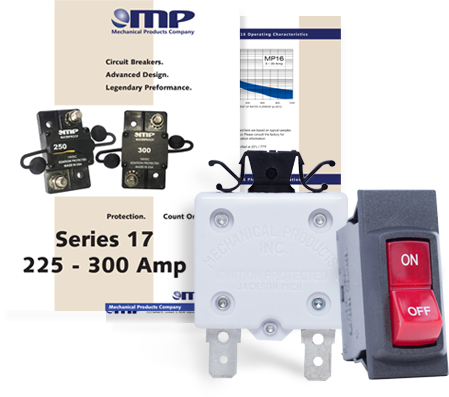## Latest MP Articles